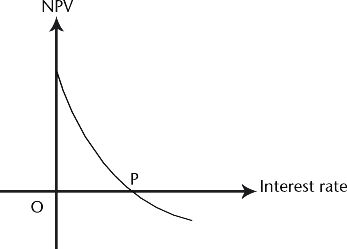# What is the internal rate of return (IRR) of a project in term of the Net Present value (NPV)?

The IRR of a project is the rate at which the NPV is zero or the present value of costs and benefits are equal or the Benefit-cost ratio is one.

In the figure below displaying the relationship between the NPV and the interest rate, the point P is the maximum interest rate at which it would be economical to undertake the project.